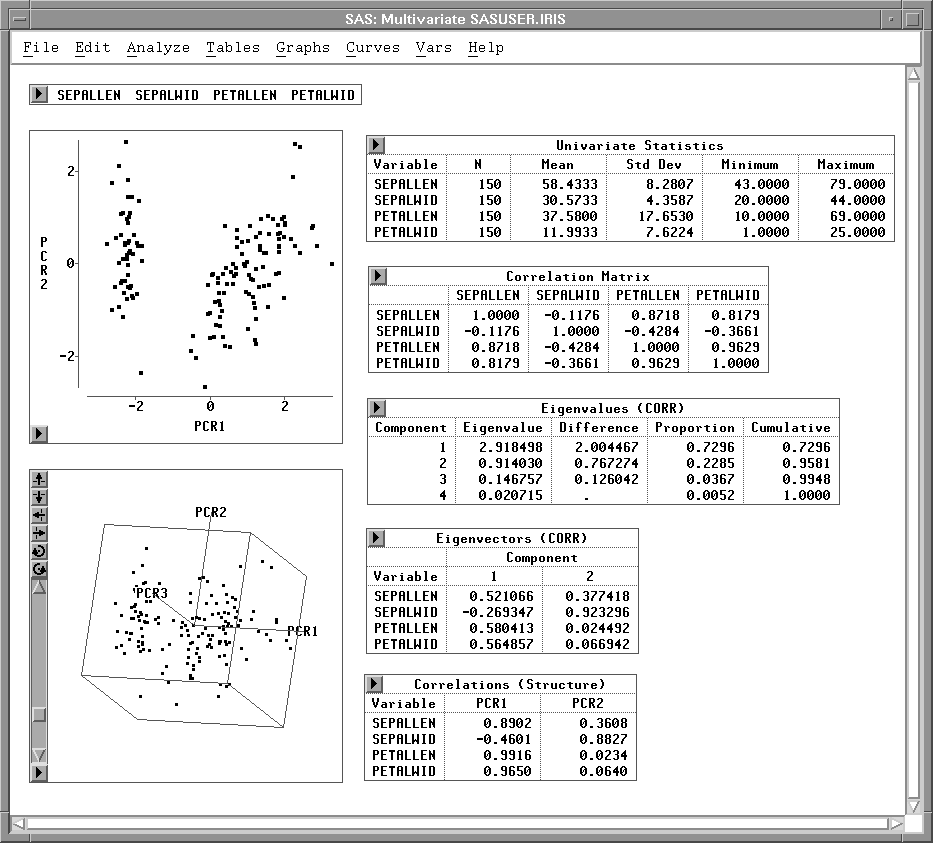Previous Page Next Page
 Multivariate Analyses

# Multivariate Analyses

Choosing Analyze:Multivariate ( Y X ) gives you access to a variety of multivariate analyses. These provide methods for examining relationships among variables and between two sets of variables.

You can calculate correlation matrices and scatter plot matrices with confidence ellipses to explore relationships among pairs of variables. You can use principal component analysis to examine relationships among several variables, canonical correlation analysis and maximum redundancy analysis to examine relationships between two sets of interval variables, and canonical discriminant analysis to examine relationships between a nominal variable and a set of interval variables.Figure 40.1: Multivariate Analysis

#### References

 Previous Page Next Page Top of Page